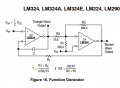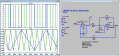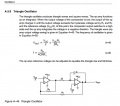# Onsemi's LM324 Function Generator question

#### Fran3

Joined Mar 28, 2019
45
Shown below is the Function Generator schematic, as illustrated in Figure 16 of the Onsemi LM324 OpAmp datasheet ...Note in the frequency equation given the reference to R(c) ...
However... there is no R(c) in the schematic so...
What does Rc represent?
I know there are other different designs for LM324 function generators but I was curious with what was going on with this one.
Thanks for any help.PS - At first I guessed R(c) may be their way of referencing X(c) but then why wouldn't they just put X(c) in the equation?

#### KeithWalker

Joined Jul 10, 2017
2,919
The equivalent circuit of a capacitor contains both series and parallel resistance. See the attached url.
In this case, Rc refers to the series resistance which is usually quite small and can be ignored in the formula in most cases because its value is insignificant when compared to R1.

#### Papabravo

Joined Feb 24, 2006
20,584
Looks like a typo. I believe that if you use the value of R2 (300K) in place of Rc you will get the correct result.
For example: If C = 1μF and Rf=10K and Rc=R2=300K you should get:

$$f\;=\;\cfrac{R1+R2}{4CR_fR1}\;=\;\cfrac{100K+300K}{4(1\times 10^{-6})(10K)(100K)}\;=\;\cfrac{400,000}{4000}\;=\;100 \text{ Hz.}$$

This of course depends on R3 = R1 || R2. This result is consistent with a simulation of the circuit.

Last edited:
•k1ng 1337 and MisterBill2

#### Papabravo

Joined Feb 24, 2006
20,584
The equivalent circuit of a capacitor contains both series and parallel resistance. See the attached url.
In this case, Rc refers to the series resistance which is usually quite small and can be ignored in the formula in most cases because its value is insignificant when compared to R1.
Certainly plausible. Is that explanation compatible with a simulation of the circuit assuming Rc can be neglected? I think perhaps not.The frequency ratio is consistent with a resistor ratio of 4.7:1

Last edited:
•k1ng 1337

#### Audioguru again

Joined Oct 21, 2019
6,432
An old LM324 quad and LM358 dual opamp produce crossover distortion that can be seen on triangle and sine waveforms.
They are also very noisy (hiss) and have slew rate limiting of high levels of frequencies above only 2kHz.

All audio opamps are low noise, produce very low distortion and work perfectly up to at least 100kHz.

#### Papabravo

Joined Feb 24, 2006
20,584
An old LM324 quad and LM358 dual opamp produce crossover distortion that can be seen on triangle and sine waveforms.
They are also very noisy (hiss) and have slew rate limiting of high levels of frequencies above only 2kHz.

All audio opamps are low noise, produce very low distortion and work perfectly up to at least 100kHz.
No argument from me.

#### Ian0

Joined Aug 7, 2020
8,935
I don’t think that an “audio” op-amp is the answer here - most audio op-amps are not rail-to-rail, do not clip symmetrically, need higher supply voltages and typically have high bias currents.
You certainly need a better op-amp than an LM358, but it does need a symmetrical output with known output voltages to make a symmetrical waveform which corresponds to the equation without having to factor in the positive and negative clipping voltages separately.
I‘d recommend a rail-to-rail device for that reason. You don’t need particularly low noise, (in the teens of nV/√Hz is plenty good enough) and if you want a high output frequency, you need a good slew-rate. Conversely, if you want to generate low frequencies, a low bias current is important.

#### AnalogKid

Joined Aug 1, 2013
10,775
Here is a clip fro Op Amps For Everyone by Ron Mancini at TI. (He used to hang around here sometimes.) Here is the original:

http://web.mit.edu/6.101/www/reference/op_amps_everyone.pdf

It is essentially the same circuit with the two sections transposed. The square wave is available at the R-Rf node, the output of the first opamp.

The circuit in post #1 is a bit more complicated because of the way it handles hysteresis.

This circuit assumes that the output of the first opamp is perfectly rail-to-rail, which it almost certainly is not. This means the actual frequency will be a bit less than calculated.

To use this circuit, first select R1 and Rf to set the output amplitude. When you plug these values into the frequency equation, you have one equation with three unknowns: Frequency, R, and C. A typical process would be to know what frequency you wand and what capacitors you have on hand, re-arrange the f equation, and calculate the timing resistor R for any particular capacitor value.

akLast edited:

#### Fran3

Joined Mar 28, 2019
45
In this thread Ian0 & others suggested the LM324/LM358 were not the best choice due to cross-over distortion and not having rail-to-rail performance.
What "rail-to-rail" Single Supply OpAmp instead of the LM324 or LM358?
Thanks for the help.

#### Ian0

Joined Aug 7, 2020
8,935
Look at Microchip's MCP60xy, where y is the number of op-amps in the package and x is the speed, but they only work up to 5V supply.
Or go to https://www.ti.com/amplifier-circuit/op-amps/products.html and tick the boxes for rail-to-rail IN and OUT.
One proviso: The second op-amp in your circuit is being used as a comparator. Many op-amps will operate properly as comparators, but not all of them!

#### AnalogKid

Joined Aug 1, 2013
10,775

#### sparky 1

Joined Nov 3, 2018
742
Last edited: